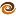# EmptyCrate

#### About Me | Contact Me | Training |ChaiScript.com | YouTube Channel

The first Project Euler problem is to calculate the sum of all integers below 1000 which are divisible by either 3 or 5. My solution is implemented entirely in C++ templates. The value is recursively calculated at compile time. The template specialization `struct Problem1<0>` stops the recursion and returns 0. To compile this code with gcc you must expand the maximum allowed template recursion depth to at least 1000. ` g++ EulerProblem1.cpp -ftemplate-depth-1000`

``````//EulerProblem1.cpp
#include <iostream>

template struct Problem1 {
static const int value = (((count % 3) == 0 || (count % 5) == 0)?count:0) + Problem1::value;
};

template<>
struct Problem1<0> {
static const int value = 0;
};

int main() {
std::cout << Problem1<999>::value << std::endl;
}
``````
My Videos

Related